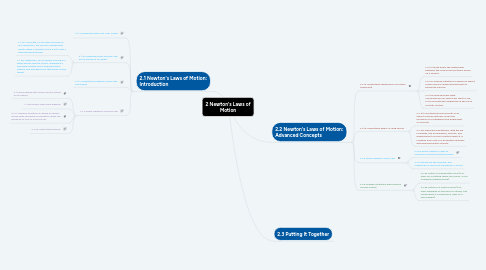# 2 Newton's Laws of MotionJennifer Dick
Get Started. It's Free2 Newton's Laws of Motion## 1. 2.1 Newton's Laws of Motion: Introduction

### 1.2. 2.1.1b Understand the Second Law: Force acting on an object

1.2.1. 2.1.1b1 Calculate, for an object moving in one dimension, the velocity change that results when a constant force F acts over a specified time interval

1.2.2. 2.1.1b2 Determine, for an object moving in a plane whose velocity vector undergoes a specified change over a specified time interval, the average force that acted on the object.

### 1.4. 2.1.2 Apply Newton’s Second Law

1.4.1. 2.1.2a Recognize the various forces acting on an object

1.4.2. 2.1.2b Draw a free-body diagram

1.4.3. 2.1.2c Analyze situations in which an object moves with specified acceleration under the influence of one or more forces

1.4.4. 2.2.2a Understand tension

## 2. 2.2 Newton's Laws of Motion: Advanced Concepts

### 2.1. 2.2.1a Understand significance of friction coefficient

2.1.1. 2.2.1a1 Write down the relationship between the normal and frictional forces on a surface.

2.1.2. 2.2.1a2 Analyze situations in which an object moves along a rough inclined plane or horizontal surface.

2.1.3. 2.2.1a3 Analyze under what circumstances an object will start to slip, or to calculate the magnitude of the force of static friction.

### 2.2. 2.2.1b Understand effect of drag forces

2.2.1. 2.2.1b1 Find the terminal velocity of an object moving vertically under the influence of a retarding force dependent on velocity.

2.2.2. 2.2.1b2 Describe qualitatively, with the aid of graphs, the acceleration, velocity, and displacement of such a particle when it is released from rest or is projected vertically with specified initial velocity.

### 2.3. 2.2.2 Apply Newton’s Third Law

2.3.1. 2.2.2b Apply Newton's laws to scenarios involving multiple bodies

2.3.2. 2.2.2 Determine the direction and magnitude of the force exerted by a spring

### 2.4. 2.2.3 Analyze situations with uniform circular motion

2.4.1. 2.2.3a Motion in a horizontal circle (e.g., mass on a rotating merry-go-round, or car rounding a banked curve).

2.4.2. 2.2.3b Motion in a vertical circle (e.g., mass swinging on the end of a string, cart rolling down a curved track, rider on a Ferris wheel).